# Length - math word problems

1. Steps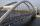Patrick step is 65 cm long and his dad step is 10 cm longer. Dad goes bridge in 52 steps. How many steps will go the same bridge by Patrick?
2. Good swimmerGood swimmer swims 23 m distance with ten shots. With how many shots he swim to an island located 81 m if still swims at the same speed?
3. Cycling trip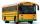At 8 am started a group of children from the camp to day cycling trip. After 9:00 weather deteriorated sharply and the leader decided to send for the children bus on the same route, which started at 10 hours. For how long and at what distance from the cam
4. Surface and volume od cuboidContent area of the square base of cuboid is Sp = 36 cm2 and its height 80 mm. Determine its surface area and volume.
5. CanisterGasoline is stored in a cuboid canister having dimensions 44.5 cm, 30 cm, 16 cm. What is the total weight of a full canister when one cubic meter of gasoline weighs 710 kg and the weight of empty canister is 1.5 kg?
6. Tetrahedral pyramidIt is given a regular tetrahedral pyramid with base edge 6 cm and the height of the pyramid 10 cm. Calculate the length of its side edges.
7. Sunflower Field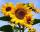The trapezoidal sunflower field is located between two parallel paths which are spaced 230 meters apart. The lengths of the parallel sides of the field are 255 m and 274 m. How many tons of sunflower will come from this field if the hectare yield is 2.25
8. Rectangular triangle PQRIn the rectangular triangle PQR, the PQ leg is divided by the X point into two segments of which longer is 25cm long. The second leg PR has a length 16 cm. The length of the RX is 20 cm. Calculate the length p of side RQ. The result is round to 2 decima
9. Axial cutThe cone surface is 388.84 cm2, the axial cut is an equilateral triangle. Find the cone volume.
10. Rotating coneCalculate the volume and the surface area of a rotating cone of base radius r = 2.3 dm and a height h = 46 mm.
11. PyramidThe pyramid has a base rectangle with a = 6cm, b = 8cm. The side edges are the same and their length = 12.5 cm. Calculate the surface of the pyramid.
12. Hexagonal pyramidRegular hexagonal pyramid has dimensions: length edge of the base a = 1.8 dm and the height of the pyramid = 2.4 dm. Calculate the surface area and volume of a pyramid.
13. Regular triangular prismCalculate the surface area of body of regular triangular prism, when the length of its base edge is 6.5 cm and height 0.2 m.What is the diameter of a tree around which is 3m 9dm 7cm long rope wrapped? How big is its cross-sectional area?
15. Scale of mapJames travels one kilometer in 12 minutes. The route walked for half an hour measured on the map 5 cm. Calculate how many kilometers James walked for half an hour. Find the scale of the map.
16. RectangleThe length of the rectangle is 12 cm greater than 3 times its width. What dimensions and area this rectangle has if ts circumference is 104 cm.
17. Engine pulley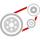The engine has a 1460 rev / min (RPM). Disc diameter is 350 mm. What will be the disc peripheral speed in RPM? Pulleys on the engine has diameter 80mm, on a disc has diameter 160mm.
18. Copper plateCalculate the thickness of the copper plate with a density 8.7 g/cm³ measuring 1.5 meters and 80 cm and its weight is 3.65 kg
19. Call of Duty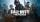Game Call of Duty in CTF (Capture the Flag) opposes the British and the Germans, whose bases are 15 km apart. A German soldier stole the British flag and hid it with it in a secret place between the bases. If the British soldiers set out at a speed of 14
20. Fence of the garden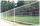The garden has dimensions of 5m and 400cm. How many meters meshes are needed for fencing the plot?

Do you have an interesting mathematical word problem that you can't solve it? Submit math problem, and we can try to solve it.

We will send a solution to your e-mail address. Solved examples are also published here. Please enter the e-mail correctly and check whether you don't have a full mailbox.

Please do not submit problems from current active competitions such as Mathematical Olympiad, correspondence seminars etc...

Do you want to convert length units?